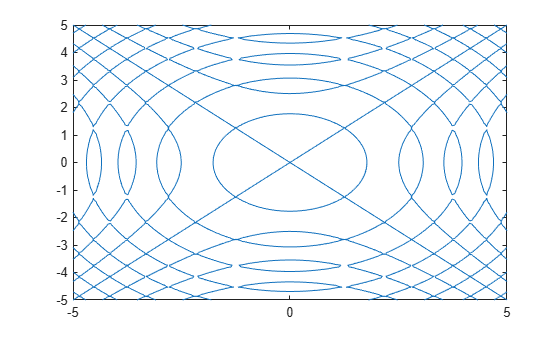# eq

Define symbolic equation

## Syntax

``A == B``
``eq(A,B)``

## Description

example

````A == B` defines a symbolic equation. Use the equation as input to functions such as `solve`, `assume`, `fcontour`, and `subs`.```
````eq(A,B)` is equivalent to `A == B`.```

## Examples

collapse all

Solve this trigonometric equation. Define the equation by using the `==` operator.

```syms x eqn = sin(x) == cos(x); solve(eqn,x)```
```ans = pi/4```

Plot the equation $\mathrm{sin}\left({x}^{2}\right)=\mathrm{sin}\left({y}^{2}\right)$ by using `fimplicit`. Define the equation by using the `==` operator.

```syms x y eqn = sin(x^2) == sin(y^2); fimplicit(eqn)```Test the equality of two symbolic expressions by using `isAlways`.

```syms x eqn = x+1 == x+1; isAlways(eqn)```
```ans = logical 1```
```eqn = sin(x)/cos(x) == tan(x); isAlways(eqn)```
```ans = logical 1```

Check the equality of two symbolic matrices by using `isAlways`.

```A = sym(hilb(3)); B = sym([1 1/2 5; 1/2 2 1/4; 1/3 1/8 1/5]); isAlways(A == B)```
```ans = 3×3 logical array 1 1 0 1 0 1 1 0 1```

Compare a matrix and a scalar. The `==` operator expands the scalar into a matrix of the same dimensions as the input matrix.

```A = sym(hilb(3)); B = sym(1/2); isAlways(A == B)```
```ans = 3×3 logical array 0 1 0 1 0 0 0 0 0```

## Input Arguments

collapse all

Input, specified as a number, vector, matrix, or array, or a symbolic number, scalar variable, matrix variable, array, function, matrix function, or expression.

Input, specified as a number, vector, matrix, or array, or a symbolic number, scalar variable, matrix variable, array, function, matrix function, or expression.

## Tips

• Calling `==` or `eq` for nonsymbolic `A` and `B` invokes the MATLAB® `eq` function. This function returns a logical array with elements set to logical `1 (true)` where `A` and `B` are equal; otherwise, it returns logical `0 (false)`.

• If both `A` and `B` are arrays, then they must have the same dimensions. `A == B` returns an array of equations `A(i,j,...) == B(i,j,...)`.

## Version History

Introduced in R2012a

expand all